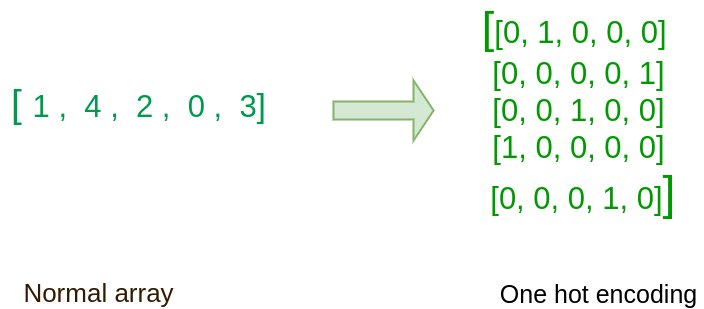Related Articles
One Hot Encoding using Tensorflow
• Difficulty Level : Medium
• Last Updated : 02 Sep, 2020

In this post, we will be seeing how to initialize a vector in TensorFlow with all zeros or ones. The function you will be calling is `tf.ones()`. To initialize with zeros you could use `tf.zeros()` instead. These functions take in a shape and return an array full of zeros and ones accordingly.

Code:

 `import` `tensorflow as tf ` ` `  `ones_matrix ``=` `tf.ones([``2``, ``3``]) ` `sess ``=` `tf.Session() ` `ones ``=` `sess.run(ones_matrix) ` `sess.close() ` ` `  `print``(ones) `

Output:

`[[1. 1. 1.]  [1. 1. 1.]]`

Using One Hot Encoding:
Many times in deep learning and general vector computations you will have a y vector with numbers ranging from 0 to C-1 and you want to do the following conversion. If C is for example 5, then you might have the following y vector which you will need to convert as follows:One Hot Encoding Example

This can be done as follows:

Parameters passed to the function:

indices: A Tensor of indices.
depth: A scalar defining the depth of the one hot dimension.
on_value: A scalar defining the value to fill in output when indices[j] = i. (default : 1)
off_value: A scalar defining the value to fill in output when indices[j] != i. (default : 0)
axis: The axis to fill (default : -1, a new inner-most axis).
dtype: The data type of the output tensor.
name: A name for the operation (optional).

Code:

 `indices ``=` `[``1``, ``4``, ``2``, ``0``, ``3``] ` `C ``=` `tf.constant(``5``, name ``=` `"C"``) ` `     `  `one_hot_matrix ``=` `tf.one_hot( ` `    ``indices, C, on_value ``=` `1.0``, off_value ``=` `0.0``, axis ``=``-``1``) ` ` `  `sess ``=` `tf.Session() ` ` `  `one_hot ``=` `sess.run(one_hot_matrix) ` ` `  `sess.close() ` ` `  `# output is of dimension 5 x 5 ` `print``(one_hot)`

Output:

```[[0.0, 1.0, 0.0, 0.0, 0.0 ]

[0.0, 0.0, 0.0, 0.0, 1.0]

[0.0, 0.0, 1.0, 0.0, 0.0]

[1.0, 0.0, 0.0, 0.0, 0.0]

[0.0, 0.0, 0.0, 1.0, 0.0]]```

Feel free to change values and see the result.

Code:

 `indices ``=` `[[``0``, ``2``], [``1``, ``-``1``]] ` `C ``=` `tf.constant(``5``, name ``=` `"C"``) ` `     `  `one_hot_matrix ``=` `tf.one_hot( ` `    ``indices, C, on_value ``=` `1.0``, off_value ``=` `0.0``, axis ``=``-``1``) ` ` `  `sess ``=` `tf.Session() ` ` `  `one_hot ``=` `sess.run(one_hot_matrix) ` ` `  `sess.close() ` `     `  `# output is of dimension 2 x 2 x 3 ` `print``(one_hot)  `

Output :

```[[[1.0, 0.0, 0.0],

[0.0, 0.0, 1.0]],

[[0.0, 1.0, 0.0],

[0.0, 0.0, 0.0]]] ```My Personal Notes arrow_drop_up
Recommended Articles
Page :